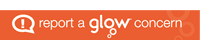# UNIT 2 – RELATIONSHIPS

Straight Line

Functional Notation f(x)

Linear Equations

Simultaneous Equations

Changing the Subject of a Formula

Pythagoras II – Problem Solving and Use in 3D

Angles and Polygons

Angles and Circles

Similarity

Trigonometric Graphs

Trigonometric Equations